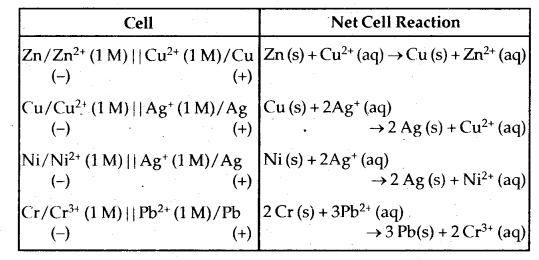# Bihar Board Class 12 Chemistry Solutions Chapter 3 Electrochemistry

Bihar Board Class 12 Chemistry Solutions Chapter 3 Electrochemistry Textbook Questions and Answers, Additional Important Questions, Notes.

Electrochemistry Class 12 NCERT Solutions help to score more marks.

## BSEB Bihar Board Class 12 Chemistry Solutions Chapter 3 Electrochemistry

### Bihar Board Class 12 Chemistry Electrochemistry Intext Questions and Answers

Question 1.
How would you determine the standard electrode potential of the system Mg2+/Mg?
The electrode of Mg dipping in the solution of its own ions Mg2+ is connected to Standard Hydrogen electrode (SHE) through a salt bridge internally and through voltmeter in the outer circuit to complete the cell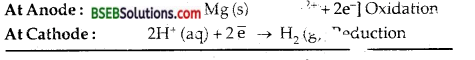Cell reaction is Mg (s) + 2H+ (aq) → Mg2+ (aq) + H2 M. The e.m.f. of the ceil is determined which is 2.36 V.
Since SHE acts as cathod
∴ According to conventiion, the standar electrode pen Mg2+/Mg system is – 2.36 V.

Question 2.
Can you store copper sulphate solution.
In this problem, we want to see whether the tone takes place or not?
CuSO4 + Zn → ZnSO4 + Cu i.e.,
Zn (s) + Cu2+ → Zn2+ + Cu (s)

By convention, the cell maybe represented by
Zn (s) | Zn2+|| Cu2+ Cu (s)
EZn2+/Zn= -0.76 V
ECu2+/Cu = +0.34 V
E0Cell = E0R.H.S – E0L.H.S
= +0.34-(-0.76) = 1.1 V
Since the e.m.f. comes out to be positive, it implies that the reaction between CuSO4 and zinc will take place.
Hence CuSO4 solution cannot be stored in a zinc pot.

Question 3.
Consult the table of standard electrode potentials and suggest three substances that can oxidize ferrous ions under suitable conditions.
Fe2+ → Fe3+ + e oxidation
$$\frac{1}{2}$$ cl2 (g) + e → Cl ] reduction
Substances like F2, Cl2, Br2 which have more values of standard reduction potentials than that of Fe3+ [0.77V] will be able to oxidize Fe2+ ions to Fe3+ ions.

Question 4.
Calculate the potential of hydrogen electrode in contact with a solution where pH is 10.
pH = 10
∴ [H+] – 1 × 10-10
H+ + e → H2(g)
Applying Nemst’s equation, we get

EH+/H2 = $$\mathrm{E}_{\mathrm{H}^{+} / \mathrm{H}_{2}}^{\circ}-\frac{0.0591}{1} \log \frac{1}{\left[\mathrm{H}^{+}\right]}$$
E = 0 + 0.0591 log [H+] [E0 of NHE = 0]
= 0.0591 × log 10-10 [log 10 = 1
= 0.0591 (-10) log 10
EH+/H2 = -0.591 v

Question 5.
Calculate the emf of the cell in which the following reaction takes place. Given Eθcell = 1.05 V.
Ni (s) + 2 Ag+ (0.002 M) → Ni2+ (0.160 M) + 2 Ag (s)
The probable cell isEcell = E0cell – $$\frac{0.0591}{\mathrm{n}} \log \frac{\left[\mathrm{Ni}^{2+}\right][\mathrm{Ag}]^{2}}{[\mathrm{Ni}]\left[\mathrm{Ag}^{+}\right]^{2}}$$
Here n = 2
Ecell = E0cell – $$\frac{0.0591}{2} \log \frac{\left[\mathrm{Ni}^{2+}\right]}{\left[\mathrm{Ag}^{+}\right]^{2}}$$
[ ∵ [Ni] = 1,[Ag] = 1]
Ecell = $$1.05-\frac{0.0591}{2} \log \frac{0.16}{(.002)^{2}}$$
Ecell = 0.91V.Question 6.
The cell in which the following reaction occurs 2 Fe3+ (aq) + 2I (aq) → 2 Fe2+ + I2 (s) has E°cell = 0.236 V at 298 K. Calculate the standard Gibbs energy and the equilibrium constant of the cell reaction.
ΔrGθ = – nFEe = -2 × 96500 × 0.236 × 103 kj mol-1
= – 45.6 kj mol-1.
cell = $$\frac{0.0591}{2} \log \mathrm{K}_{\mathrm{c}}$$
∴ $$\frac{0.236 \times 2}{.0591}$$ = log Kc
∴ Kc= antilog 7.9864 = 9.7 × 107.

Question 7.
Why does the conductivity of a solution decrease with dilution?
The conductivity of an electrolyte falls with dilution because the number of current-carrying particles, i.e., ions present per cm3 of the solution becomes less and less on dilution.

Question 8.
Suggest a way to determine the Am of water.
Water is a very weak electrolyte. The value of Am of water can be determined using Kohlrausch’s law.
A0m(H2O) = $$\lambda_{\mathrm{m}\left(\mathrm{H}^{+}\right)}^{\circ}+\lambda^{\circ} \mathrm{m}\left(\mathrm{OH}^{-}\right)$$
= [λ0m(H+)]+[λ0m(OH)]
= A0m(HCl) + A0m(NaOH) – A0m(NaCl)

Question 9.
The molar conductivity of 0.025 mol L-1 methanoic acid is 46.1 S cm2mol-1. Calculate its degree of dissociation and dissociation constant. Given λ0H+ = 349.6 S cm2 mol-1 and λ0(HCOO)= 54.6 S cm2mol-1.
Degree of dissociation (a) of methanoic aid =$$\frac{\Delta_{\mathrm{c}}}{\Delta^{\circ}}=\frac{\Delta_{\mathrm{C}}}{\lambda_{\mathrm{H}^{+}}^{\circ}+\lambda_{\mathrm{HCOO}^{-}}^{0}}$$
= $$\frac{46.1}{349.6+54.6}=\frac{46.1}{404.2}$$ = 0.114

Dissociation constant of methanoic acid
= $$\frac{C \alpha^{2}}{1-\alpha}$$
= $$\frac{0.025 \times(0.114)^{2}}{1-0.114}$$
= $$\frac{0.025 \times(0.114)^{2}}{0.886}$$
= $$\frac{0.025 \times 0.012996}{0.886}$$
= 3.67 × 10-4 mol L-1.

Question 10.
If a current of 0.5 ampere flows through a metallic wire for 2 hours, then how many electrons flow through the wire?
Q = I × t = 0.5 × 2 × 60 × 60 C
= 3600 C
Charge present on 1 electron = 1.6023 × 10-19 C
∴ No. of electrons flowing = $$\frac{3600}{1.6023 \times 10^{-19}}$$
= 2.25 × 1022
or No. of Moles of electrons = $$\frac{2.25 \times 10^{22}}{6.023 \times 10^{23}}$$ = 3.73 × 10-2.

Question 11.
Suggest a list of metals that are extracted electrolytically.
Metals like Li, Na, K, Mg, Ca which are strong reducing agents themselves can’t be extracted by reducing their salts with other reducing agents.
∴ They are extracted from their molten solutions by electrolysis.

Question 12.
Consider the reaction:
Cr2O2-7 + 14H+ + 6e → 2 Cr3+ + 8H2O
What is the quantity of electricity in coulombs needed to reduce 1 mol of Cr2O2-7?
For reducing 1 mole of Cr2O2-7 ions 6 moles of electrons are required. For 1 mole of electrons ‘1F’ or 96,500 C of electricity is required.
∴ For passing 6 moles of electrons or 6F of electricity is required = 6 × 96,500 C = 579,000 C.Question 13.
Write the chemistry of recharging the lead storage battery, highlighting all the materials that are involved during recharging.
During recharging the cell is operated like an electrolytic cell, i.e., now electrical energy is supplied to it from an external source. The electrode reactions are the reverse of those that occur during discharge.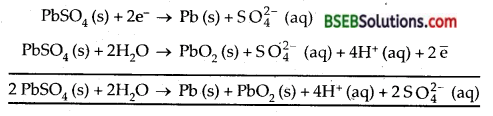Such operation is possible because PbSO4 formed during discharge is a solid and sticks to the electrodes. It is, therefore, in a position to either receive or give up electrons during electrolysis.

Question 14.
Suggest two materials other than hydrogen that can be used as fuels in fuel cells.
Two other substances which can he used as materials in fuel cells other than hydrogen are CO, methane (CH4) and methanol (CH3OH).

Question 15.
Explain how rusting of iron is envisaged as setting up of an electrochemical cell.
The rusting of iron in the presence of water and air is essentially an electrochemical phenomenon. A series oI tiny electrochemical cells are set upon the surface of iron.
Anode: 2 Fe (s) → 2Fe2+ + 4e Fe2+/Fe = -0.44 V
Electrons are lost at anode Here Fe is oxidised to Fe2+. Electrons thus lost at anodic spot move through the metal and reduce oxygen in the presence of H+ ions.

Cathode: O2 +4H++4e → 2H2O(l); E°H+|O2|H2O
The overall reaction is
2 Fe (s) + O2 (g) + 4H+ (aq) → 2Fe2+ + 2H2O (l); E°cell = 1.67 V
Atmospheric Oxidation takes place when Fe2+ ions are oxidized to Fe2O3 by oxygen of air
2 Fe2+ (aq) + 2 H2O (l) + $$\frac{1}{2}$$O2(g) → Fe2O3 (s) + 4H+ (aq).### Bihar Board Class 12 Chemistry Electrochemistry Text Book Questions and Answers

Question 1.
Arrange the following metals in the order in which they displace each other from the solution of their salts.
Al, Cu, Fe, Mg and Zn.
The standard reduction of these metals are – 1.66 V, + 0.34 V, – 0.44 V, – 2.37 V and – 0.76 respectively.
The lower the reduction potential, more the tendency to lose electrons and thus more will be the displacement power. Thus the decreasing order of displacement power is Mg > Al > Zn > Fe > Cu.

Question 2.
Given the standard electrode potentials. K+/K = -2.93 V,Ag+/Ag = 0.80 V, Hg2+/Hg = 0.79 V
Mg2+/Mg = – 2.37 V, Cr3+\Cr = – 0.74 V.
Arrange these metals in their increasing order of reducing power.
The reducing power of a metal depends upon its tendency to lose Electrons. Thus lower the reduction potential, more the tendency to get oxidized and thus more will be the reducing power. Hence the increasing order of their reducing power is Al < Hg < Cr < Mg < K.

Question 3.
Depict the galvanic cell in which the reaction Zn (s) + 2 Ag+ (aq) → Zn2+ (aq) + 2 Ag (s) takes place. Further show:
(i) Which of the electrode is negatively charged?
(ii) The carriers of the current in the cell.
(iii) Individual reaction at each electrode.
The cell reaction is Zn (s) + 2 Ag+ (aq) → Zn+2 (aq) + 2 Ag.
The reaction occuring at ANODE is
Zn (s) → Zn++ + 2e] oxidation half reaction
The reaction occuring at CATHODE is
2 Ag+ (aq) + 2e → 2 Ag (s)] reduction half reaction .

The complete cell reaction is
Zn (s) + 2 Ag+ (aq) → Zn2+ + 2 Ag (s)
The representation of the cell will be
Zn (s) |Zn++ (aq) || Ag+ (aq) | Ag (s)
N.D. Study Material Based On Depiction of the Galvanic CellElectrons flow from anode to cathode in the outer circuit. Conventional current flow against the flow of electrons from cathode to anode. Anode is given the negative sign and cathode is given the positive.

Question 4.
Calculate the standard cell potentials of galvanic cell in which the following reactions take place:
(i) 2 Cr (s) + 3 Cd2+ (aq) → 2 Cr3+ (aq) + 3 Cd
(ii) Fe2+ (aq) + Ag+ (aq) →Fe3+ (aq) + Ag (s)
Calculate the ΔrGθ and equilibrium constant of the reactions.
The galvanic cell may be represented as Cr (s) | Cr3+ (aq) || Cd2+ (aq) | Cd (s)
Reaction occuring at anode
Cr (s) → Cr3+ + 3e] × 2
oxidation half reaction
Reaction occuring at cathode
Cd2+ + 2e → Cd (s) ] × 3
reduction half reaction Adding the two half reactions;
cell reaction is
2 Cr (s) + 3 Cd2+ (aq) → 2 Cr3+ (aq) + 3Cd (s)
E0cell = E0Red[R-H.S.]-E°Red[L.H.S.]
E0Cd2+,Cd – E0Cr3+,Cr
= – 0.40 – (- 0.74) = + 0.34 VOLT.

ΔrGθ = -nF E0cell
Here n = 6 F= 96,500 C
E0cell = +0.34 V
ΔrGθ = -6 × 96500 × 0.34
= -1.968 ×105J [1C × 1 V = 1J]

Calculation Of K
ΔrGθ = – 2.303 RT log K 1
= – 2.303 × 8.314 × 298 log K
[∵R = 8.314 JK-1 Mol-1 T = 298 K]
Now ΔrGθ = -1.968 × 105 J(Calculated above)
∴ -1.968 × 105 = – 2.303 × 8.314 × 298 log K
log K = $$\frac{1.968 \times 10^{5}}{2.303 \times 8.314 \times 298}$$
K = antilog 34.501 = 3.17 x 1034

(ii) The galvanic cell may be represented by
Fe2+ (aq) |Fe3+ (aq) || Ag+ (aq) | Ag (s)
The reaction at anode
Fe2+→ Fe3+ + e ] Oxidation half reaction

The reaction at cathode
Ag+ (aq) + e → Ag (s) ] Reduction half reaction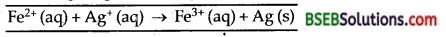cell = E0Red[R.H.S.]-E0Red[L.H.S.]
= E°Ag+,Ag – E°Fe2+,Fe3+
= (0.80-0.77) = 0.03 V
ΔrGθ= – n F E°cell [n = 1, F = 96,500 C]
cell = -(1) (96500) (0.03)
= – 2895 J mol-1

ΔrGθ = – 2895 J = -2.895 KJ mol-1
ΔrGθ = – 2.303 RT log K
log K = –$$\frac{\Delta_{\mathrm{r}} \mathrm{G}^{\theta}}{2.303 \mathrm{RT}}$$
= – $$\frac{-2895}{2.303 \times 8.314 \times 298}$$
= 0.5074
K = antilog 0.5074 = 3.217Question 5.
Write the Nemst equation and emf of the following cells at 298 K:
(i) Mg (s) | Mg2+ (0.001 M) || Cu2+ (0.0001 M)| Cu (s)
(ii) Fe (s) | Fe2+ (0.001 M) |] H+ (1M) | H2 (g) (1 bar) | Pt (s)
(iii) Sn (s) | Sn2+ (0.050 M) ||H+ (0.020 M) | H2 (g) (1 bar) | Pt (s)
(iv) Pt (s) | Br2 (l) | Br (0.010 M) ||H+ (0.030 M) | H2 (g) (1 bar) | Pt (s).
Mg (s) | Mg2+ (.001 M) || Cu2+ (0.0001 M) | Cu (s)
Reduction reaction are
Mg+2 + 2e → Mg(s)
Cu+2 + 2e → Cu (s)
Cell reaction is
Mg (s) + Cu++ → Mg++ + Cu (s)

Ecell = E°cell – $$\frac{\mathrm{RT}}{\mathrm{nF}} \ln \frac{\left[\mathrm{Mg}^{+2}\right][\mathrm{Cu}]}{[\mathrm{Mg}]\left[\mathrm{Cu}^{++}\right]}$$
Ecell = E°cell – $$\frac{\mathrm{RT}}{\mathrm{nF}} \ln \frac{\left[\mathrm{Mg}^{2+}\right]}{\left[\mathrm{Cu}^{2+}\right]}$$
Since [Cu] = 1
[Mg] = 1

Cu++,Cu – E°Mg+ + ,Mg
= – $$\frac{0.0591}{2} \log \frac{0.001}{0.0001}$$ [ n= 2]
= [0.34 -(-2.36)]
= 2.7 – 0.0295 log 10 [log 10 = 1]
Ecell = (2.7-0.0295) V = 2.68 V

(ii) Fe (s) | Fe2+ (0.001 M) || H+ (1 M), H2 (g) (1 bar) | Pt (s)
Cen reaction is
Fe (s) +2n → Fe2+ + H2 (g)
Ecell = E°cell – $$\frac{\mathrm{RT}}{\mathrm{nF}} \ln \frac{\left[\mathrm{Sn}^{2+}\right]}{\left[\mathrm{H}^{+}\right]^{2}}$$ [Sn(s)= 1]
= [ E° H+,H2 – E° Sn+,Sn] – $$\frac{0.0591}{2} \log \frac{0.05}{(0.02)^{2}}$$ n = 2 [Sn2+] = 0.05 M
Ecell = [0- (-0.14)]- $$\frac{0.0591}{2}$$ log 125
= 0.14 – 0.0295 × 2.0969 [H+] = 0.02 M
= 0.14 – 0.06 [ log 125 = 2.0969]
Ecell = 0.08 V

(iv) Pt (s) | Br2 (I) | Br (0.01 M) ||H+ (0.03 M) | H2 (g) (1 bar) | Pt (s)
Cell reaction is:
2 Br + 2H+ → Br2 + H2
Ecell = E°cell – $$\frac{0.0591}{2} \log \frac{1}{[\mathrm{~B} \overline{\mathrm{r}}]^{2}\left[\mathrm{H}^{+}\right]^{2}}$$
Ecell = (0 – 1.08) – $$\frac{0.0591}{2} \log \frac{1}{(0.01)^{2}(0.03)^{2}}$$
= – 1.08 – $$\frac{0.0591}{2}(7.0457)$$
=-1.08 – 0.208 = -1.288 V
Thus, oxidation will occur at the hydrogen electrode and reduction at the Br2 electrode
In that case Ecell = 1.288 V

Question 6.
In the button cells widely used in watches and other devices the following reaction takes place:
Zn (s) + Ag2O (s) + H2O (l) → Zn2+ (aq) + 2 Ag (s) + 2OH (aq) Determine ΔrGθ and Eθ for the reaction.
Given Zn (s) → Zn2+ + 2e; E° = 0.76 V ’
Ag2O + H2O + 2e → 2 Ag + 2OH;E° = 0.344 V
cell = E°Ag°2 ,Ag + E°Zn,Zn2+ )
= 0.344 + 0.76 = 1.104 V ‘
ΔrG° = -nF E°cell = – 2 × 96,500 × 1.104 J ,
= -2.13×105J

Question 7.
Define conductivity and molar conductivity for the solution of an electrolyte. Discuss their variation with concentration.
Conductivity of specific conductance (K, α : Kappa: Greek , word) is the reciprocal of resistivity. .
It is the conductance of a material which is 1 metre in length and; having an area of cross-section of 1m2. v
Its unit is Siemen (S) m-1.
1S cm-1 = 100 Sm-1
Siemen or 1 S = 1 ohm-1 or mho or Ω-1 If we take an electrolytical solution in a 1 cm3 of a vessel having: electrodes 1 cm apart, its conductance is also called specific conductance or conductivity.
Unit of K is also ohm-1 cm-1.

Molar Conductivity-It is the conducting power of all ions producing by dissolving one mole of an electrolyte in solution. It is denoted by Am (lambda). Molar conductivity is related to specific conductance/conductivity (x) as ∧m = K/M. where M is the molar concentration. If M is in the units of Molarity, i. e., moles per litre (mol L-1), ∧m may be expressed as ∧m = $$\frac{\kappa \times 1000}{\mathrm{M}}$$
Unit of Molar conductivity
Molar conductivity =’ S m2 mol-1

Specific conductance or conductivity decreases on dilution as the * number of ions per cm3 of the solution decreases on decreasing the concentration or increasing the dilution.
The variation of Molar conductance or molar conductivity with concentration can be explained on the basis of conducting ability of ions for weak and strong electrolytes.

(i) Conductivity behaviour of weak electrolytes-The variation of Am with dilution can be explained on the basis of number of ions in solution. The number of ions furnished by an electrolyte depend upon the degree of dissociation with dilution. With Increase in dilution, the degree of dissociation increases and as a result molar conductivity increases. The limiting value of molar conductance/conductivity (Δm) corresponds to degree of dissociation equal to one, i.e., the whole of the I electrolyte dissociates.

Thus the degree of dissociation can be calculated at any concentration as α = $$\frac{\Delta_{\mathrm{m}}^{\mathrm{c}}}{\Delta_{\mathrm{m}}^{\infty}}$$
where a is the degree of dissociation, Δmc is the molar conductance at infinite dilution.

Molar Conductivity of Strong Electrolytes In the case of strong electrolytes there is no increase in the number of ions with dilution because strong electrolytes are completely ionised in solution at all concentrations. However, in concentrated solution of strong electrolytes, there are strong forties of attraction between the ions of opposite charges called inter-ionic forces. Due to these inter-ionic forces, the conducting ability of the ions is less in concentrated solutions. With dilution, the ions become far apart from one another and interionic forces decrease.

As a result molar conductivity increases with dilution. When the concentration of the solution becomes very very low, the interionic attraction becomes negligible and the molar conductivity approaches the limiting value called Molar Conductivity At Infinite Dilution. It is characteristic of each electrolyte ∧m and Δm for strong electrolytes are related to each other by ∧m = Δm – b$$\sqrt{\mathrm{c}}$$;
b is a constant which depends upon the viscosity and dielectric constant of the solvent; c is the concentration of the solution.Question 8.
The conductivity of 0.20 M solution of KCl at 298 K is 0.0248 S cm-1. Calculate its molar conductivity.
K (conductivity) Molarity = 0.0248 S cm-1 = 0.20 M
Molarity = 0.20 M
m = $$\frac{\kappa \times 1000}{\text { Molarity }}$$
= $$\frac{0.0248 \mathrm{~S} \mathrm{~cm}^{-1} \times 1000 \mathrm{~cm}^{3} \mathrm{~L}^{-1}}{0.20 \mathrm{~mol} \mathrm{~L}^{-1}}$$
= 124 S cm-1 mol-1
= 124 S cm2 mol-1
∴ Molar conductivity = 124 S cm2 mol-1.Question 9.
The resistance of a conductivity cell containing 0.001 KCl solution at 298 K is 1500 Ω. What is the cell constant if conductive of 0.001 M KC1 solution at 298 K is 0.146 × 10-3 S cm-1.
Resistance of the conductivity cell = 1500 Ω
∴ Conductance = $$\frac{1}{1500}$$ ohm-1 or S
Conductivity (K) of solution = 0.146 × 10-3 S cm-1
∴ Cell Constant$$\left(\frac{l}{a}\right)$$ = $$\frac{\text { Specific conductance }}{\text { Observed conductance }}$$
= $$\frac{0.146 \times 10^{-3}}{\frac{1}{1500}}$$
= 0.146 × 10-3 × 1500 cm-1
Cell constant = 0.219 cm-1

Question 10.
The conductivity of sodium chloride at 298 K has been determined at different concentrations and the results are given below: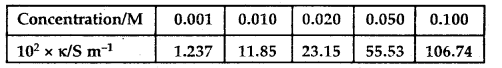Calculate Am for all concentrations and draw a plot between ∧m and c$$\frac{1}{2}$$. Find the value of ∧m0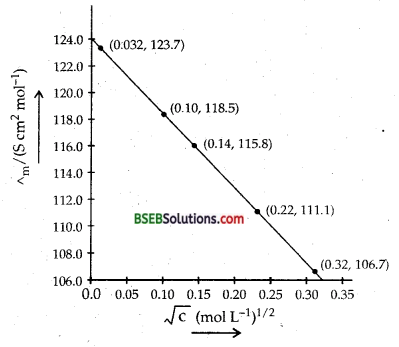∧° = intercept on Am axis = 124.0 S cm2 mol-1
on extrapolation to zero concentrationQuestion 11.
Conductivity of 0.00241 M acetic acid is 7.896 × 10-5 S cm-1. Calculate its molar conductivity and if ∧m0 for acetic acid is 390.5 S cm2 mol-1, what is its dissociation constant?
(K) Conductivity of acetic acid = 7.896 × 10-5 S cm-1
for acetic acid = 390.5 S cm2 mol-1

Δmc = $$\frac{\kappa \times 1000}{\text { Molarity }}$$
= $$\frac{7.896 \times 10^{-5} \times 1000}{0.00241}$$
= 32.76 S cm2 mol-1

α = degree of dissociation = $$\frac{\Lambda_{\mathrm{m}}^{\mathrm{c}}}{\Lambda_{\mathrm{m}}^{\circ}}=\frac{32.76}{390.5}$$ = 8.4 × 10-2
Dissociation constant of acetic acid Ka = $$\frac{\mathrm{C} \alpha^{2}}{1-\alpha}$$
= $$\frac{0.00241 \times\left(8.4 \times 10^{-2}\right)^{2}}{1-0.084}$$
= 1.86× 10-5.

Question 12.
How much charge is required for the following reductions
(i) 1 mol of Al3+ to Al.
(ii) 1 mol of Cu2+ to Cu.
(iii) 1 mol of MnO4 toMn2+.
(i) The electrode reaction is Al3+ + 3 e → Al
∴ Quantity of charge required for reduction of 1 Mole of Al3+
= 3 × F = 3 × 96500 C
= 289500 C

(ii) The electrode reaction is
Cu2+ + 2 e → Cu
Quantity of charge required for reduction of 1 Mole of Cu2+
= 2 × F = 2 × 96,500 C = 1,93,000 C

(iii) The electrode reaction is
MnO4 → Mn2+
i. e., Mn7+ + 5 e → Mn2+
Quantity of charge required, = 5F
= 5 × 96,500 C = 482500 C.Question 13.
How much electricity in terms of Faraday is required to produce
(i) 20.0 g of Ca from molten CaCl2
(ii) 40.0 g of A1 from molten Al2O3.
(i) Ca2+ + 2 e→ Ca
Thus 1 mol of Ca (= 40 g) require electrictiy = 2F
∴ 20 g of Ca will require = 1 F

(ii) Al3+ + 3 e → Al
Thus 1 mol of Al (= 27 g) require electricity = 3F
∴ 40 g of A1 will require electricity = $$\frac{3}{27}$$ × 40 = 4.4 F.

Question 14.
How much electricity is required in coulomb for the oxidation of
(i) 1 mol of H2O to O2?
(ii) 1 mol of FeO to Fe2O3?
(i) The electrode reaction for 1 mol of H2O is
H2O →H2+$$\frac{1}{2}$$ O2
i.e., O2- → $$\frac{1}{2}$$O2 + 2 e
∴ Quantity of electricity required = 2F
= 2 ×96,500 = 1,93,000 0

(ii) The electrode reaction for 1 mol of FeO is
FeO → $$\frac{1}{2}$$Fe2O3
i.e., Fe2+ → Fe3+ + e
Quantity of electricity required = F = 96,500

Question 15.
A solution of Ni(NO3)2 is electrolysed between platinum electrodes using a current of 5 amperes for 20 minutes. What mass of Ni is deposited at the cathode?
Atomic mass of N,i = 58.7
time = 20 min = 20 × 60 = 1200 sec.
Quantity of electricity passed= I × t = 5 × 1200 = 6000 C
Ni (NO3)2 will yield Ni2+ ions in the solution .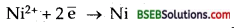Thus, 2F, i.e., 2 × 96,500 C deposit Ni = 1 Mole = 58.7 g
∴6000 C will deposit Ni = $$\frac{58.7}{2 \times 96,500}$$ × 6,000 g = 1.825 g
∴Mass of Ni deposited at cathode = 1.825 g

Question 16.
Three electrolytic cells A, B, C containing solution of ZnSO4, AgNO3 and CuSO4, respectively are connected in series. A steady current of 1.5 amperes was passed through them until 1.45 g of silver deposited at the cathode of cell B. How long did the current flow? What mass of copper and of zinc were deposited?
Atomic mass of Cu = 63.5, Zn = 65.3, Ag = 108.i.e., 108 g of Ag are deposited by 1F = 96,500 C.
∴ 1.45 g of Ag will be deposited by $$\frac{96,500}{108}$$ × 1.45 C = 1295.6 C
Q = I × t
= 14 mm, 24 sec.

Now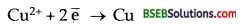i. e., 2 × 96,500 deposit Cu = 63.5
1295.6 C will deposit Cu =  × 1295.6 = 0.426 g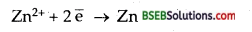∴ Zn deposited = $$\frac{65.3}{2 \times 96500} \times 1295.6$$ = 0.438 g.

Question 17.
Using the standard electrode potentials given in the Table 3.1, predict if the reaction between the following is feasible:
(i) Fe3+ (aq) and I (aq)
(ii) Ag+ (aq) and Cu (s)
(iii) Fe3+ (aq) and Br (aq)
(iv) Ag (s) and Fe3+ (aq)
(v) Br2 (aq) and Fe2+ (aq).
Given standard electrode potentials:
$$\frac{1}{2}$$ I2,I = 0.54 V E°Cu2+,Cu = 0.34
$$\frac{1}{2}$$ Br2,Br = 1.09 V E°Ag+,Ag = +0.80 V
Fe3+,Fe2+ = 0.77 V
A Reaction is feasible if EMF of the cell reaction is positive.
(i) Fe3+(aq)+I(aq) → Fe2++$$\frac{1}{2}$$+I2cell = E°Fe3+,Fe2+ – E°$$\frac{1}{2}$$I2,I
The reation is feasible = 0.77 – 0.54 = 0.23 V,

(ii) 2 Ag+ (aq) + Cu (s) →2 Ag (s) + Cu2+
cell = E°Ag+ ,Ag – E°Cu2+ ,Cu
[as the cell is Cu | Cu2+ || Ag+|Ag]
= 0.80 – 0.34 = 0.46 V

(iii) Fe3+ (aq) + Br (aq) → Fe2+ (aq) +$$\frac{1}{2}$$ Br2
cell = 0.77-1.09 = 0.32 V
The reaction is not feasible.

(iv) Ag (s) + Fe3+ (aq) → Ag+ (aq) + Fe2+ (aq)
cell = 0.77 – 0.80 = -0.03 V
The reaction is not feasible.

(v) $$\frac{1}{2}$$ Br2(aq) + Fe2+ → Br(aq)+Fe3+(aq)
cell = 1.09-0.77 = 0.32 V
The reaction is feasible.Question 18.
Predict the products of electrolysis in each of the following:
(i) An aqueous solution of AgNO3 with silver electrodes.
(ii) An aqueous solution of AgNO3 with platinum electrodes.
(iii) A dilute solution of H2SO4 with platinum electrodes.
(iv) An aqueous solution of CuCl2 with platinum electrodes.
(i) Electrolysis of aqueous solution of AgNO3 with silver electrodes
AgNO3(aq) → Ag+(aq) + NO3 (aq)
H2O ⇌ H+ + OH
At Cathode: Ag+ ions have lower discharge potential than H+ ions.

Hence Ag+ ions will be deposited in preference to H+ ions.
Ag+ + e→ Ag
At Anode: As Ag anode is attacked by NO3, Ag of the electrode will dissolve to form Ag+ ions in the solution.
Ag(s) → Ag+ + e

(ii) Electrolysis of aqueous solution of AgNO3 with platinum electrodes.
At Cathode: Same as above.
At Anode: As anode is not attackable, out of OH ions and NO3 ions, OH ions have lower discharge potential. Hence OH ions will be discharged in preference to NO3 which then decompose to give out O2
OH (aq) → OH + e
4 OH → 2H2O (l) + O2(g)

(iii) Electrolysis of dil. H2SO4 with platinum electrodes
H2SO4(aq) → 2H+(aq) + SO4-2 (aq)
H2O ⇌ H+ + OH
At Cathode: H+ + e → H
H + H →H2
At Anode: OH →OH + e
4OH → 2H2O (l) + O2 (g)

(iv) Electrolysis of aq. solution of CuCl2 with platinum electrodes
CuCl2 (s) + aq.→Cu2+ (aq) + 2 Cl (aq)
H2O ⇌ H+ + OH
At Cathode: Cu2+ ions will be reduced in preference to H+ ions.
Cu2+ + 2e → Cu (s)
At Anode: Cl ions will be oxidized in preference to OH ions.
Cl → Cl + C;Cl + Cl→Cl2(g)
Thus Cu will be deposited at cathode and Cl2 will be liberated at anode.

### Bihar Board Class 12 Chemistry Electrochemistry Additional Important Questions and Answers

Question 1.
Why Al cannot be obtained by electrolysis of fused AlCl3?
It is a covalent compound and sublimes at 453 K.

Question 2.
What is the use of platinum foil in the hydrogen electrode?
H2 gas is non-conductor. Therefore platinum foil is used for inflow and outflow of electrons.

Question 3.
How does concentration of sulphuric acid change in lead storage battery when current is drawn from it?
Concentration of sulphuric acid decreases.

Question 4.
Why does a mercury cell give a constant voltage throughout its life?
This is because the electrolytic KOH is not consumed in the reaction.

Question 5.
What are the units of cell constant?
Cell constant is $$\frac{l}{a}$$ cm-1 or metre-1.

Question 6.
Can tin coating on iron act as sacrificial anode in protecting iron against corrosion? ,
No, because tin is less readily oxidized in comparison to iron [E° Fe2+/Fe = -0.44 V; E° Sn2+/Sn = -0.14] Tin protects iron only as a cover.Question 7.
What are the products of electrolysis of (i) Molten sodium chloride (ii) aqueous sodium chloride.
Molten sodium chloride: Na, Cl2
Aqueous sodium chloride: H2 and Cl2.

Question 8.
What is the effect of decreasing concentration on the molar conductivity of weak electrolyte?
With decrease in concentration of weak electrolytes, their conductivity (molar) increases.

Question 9.
Name one cell which is generally used in space capsules?
Fuel cells of the hydrogen-oxygen type are used.

Question 10.
Write NERNST EQUATION for the following cell reaction 2 Al (s) + 3 Fe2+(aq) —> 2Al3+(aq) + 3.Fe(s)
; RT, [Al3+]2
cell =E°cell – $$\frac{\mathrm{RT}}{6 \mathrm{~F}} \ln \frac{\left[\mathrm{Al}^{3+}\right]^{2}}{\left[\mathrm{Fe}^{2+}\right]^{3}}$$

Question 11.
On electrolysis of an aqueous solution of NaCl, why H2 and not Na is liberated at the cathode?
It is because H+ ions produced from ionisation of water have lower discharge potential than Na+ ions produced from ionisation of NaCl solution.

Question 12.
How much amount of a substance is deposited by 1 coulomb? What is it called?
One coulomb deposits Eq. wt./96500 g. It is called Electrochemical equivalent of a substance.

Question 13.
Why ∧0m for acetic acid cannot be determined experimentally ?
Molar conductivity of weak electrolytes keeps on increasing with dilution and does not become constant even at very large dilutions.

Question 14.
Out of HCl and NaCl, which do you expect will have greater value for ∧0m and why?
HCl. It is because H+ ions are smaller than Na+ ions and hence H+ ions have greater ionic mobility than Na+ ions.

Question 15.
What are the products of electrolysis of molten and aqueous NaCl? (P.S.B. 1998)
(i) Na and Cl2,
(ii) H2 and Cl2.

Question 16.
Following two reactions can occur at cathode in the electrolysis of aqueous sodium chloride

Which reaction takes place preferentially and why? (A.I.S.B. 2001)
As the standard reduction potential of H2O is greater than that of Na+ ions, reduction of water takes place preferentially, i.e., H2 is liberated at cathode.

Question 17.
Copper is conducting as such while copper sulphate is conducting only in molten state or in aqueous solution. Explain.
(D.S.B. 2004, A.I.S.B. 2005)
Copper, being a metallic solid, is conducting due to mobile electrons. CuSO4 is conducting in molten or aqueous state due to mobile ions which it releases.

Question 18.
Why is it necessary to use salt bridge? (P.S.B. 2005)
To complete the electrical circuit internally and to maintain electrical neutrality of the solutions in two half cells.Question 19.
Why a cell stops working after sometime? (P.S.B. 2005)
With time, concentration of the electrolytic solutions changes. Hence their electrode potentials change. When the electrode potentials ) of the two half cells become equal, the cell stops working.

Question 20.
State the factors that influence the value of cell potential of the cell.
Mg (s) | Mg2+|| Ag+| Ag. (A.I.S.B. 2003)
Concentration of Mg2+ ions and Ag+ ions in the solution and temperature.

Question 21.
Why is the equilibrium constant K related to only E°cell and not ECell ? (DCB 2003)
It is because at equilibrium, Ecell = 0

Question 22.
Write the name of the electrolyte used in (i) fuel cell, (ii) mercury cell. (P.S.B. 2002)
(i) Concentrated aqueous KOH solution.
(ii) Moist mercuric oxide (HgO) mixed with KOH.

Question 23.
Write the names of electrodes used in a fuel -cell, (P.S.B. 2002)
Hydrogen electrode and oxygen electrode.

Question 24.
Which type of metal can be used in cathodic protection of r iron against rusting? (P.S.B. 2002)
A metal which is more electropositive than iron such as Al, Mg, Zn.

Question 25.
What would happen if the protective tin coating ovei an iron bucket is broken in some places? (D.C.B. 2002)
In such a case, iron corrodes faster than it does in the absence of tin as oxidation potential of Fe is greater than that of tin.

Question 26.
Write the reaction occurring in a fuel cell. (H.S.B. 2001)
2 H2 (g) + O2 (g) → 2H2O (l)

Question 27.
How does a fuel cell operate? (A.I.S.B. 2002)
At Anode: 2H2 (g) + 4 OH(aq) → 4H2O + 4 e ] oxidation
At Cathode : O2 (g) + 2H2O (l) + 4 e →4 OH (aq)] reduction
Cell reaction is 2H2 (g) + O2 (g) → 2H2O (l)

Question 28.
Which allotrope of carbon is used for making electrodes? (P.S.B. 1999)
Graphite.Question 29.
Write an expression to relate molar conductivity of an electrolyte to its degree of dissociation. (D.S.B. 1997, A.I.S.B. 1999)
α = ∧cm \∧0m

Question 30.
Define corrosion. What is the chemical formula of rust? (P.S.B. 1994, H.P.S.B. 96, H.S.B. 2001)
Corrosion is the slow eating away of the surface of the metal due to attack by atmospheric gases and moisture. The formula of rust is
Fe2O3. × H2O.

Question 31.
Why is chromium used for coating iron?
Chromium is a non-corroding metal which forms a protective layer on iron.

Question 32.
Can we store copper sulphate in iron vessel? Why? (H.P.S.B. 97,02)
No, because iron is more reactive than copper.

Question 33.
Name the electrolyte used in dry cell. (P.S.B. 2000,2001)
Paste of NH4Cl and ZnCl2 in the zinc cylinder and MnO2 + C surrounding the graphite rod.

Question 34.
Write Nemst equation for single electrode potential.(D.S.B. 2000, 2001)
For the electrode reaction
Mn+ + ne → M
EMn+/M = E°Mn+/M – $$\frac{2.303}{\mathrm{nF}} \log \frac{[\mathrm{M}]}{\left[\mathrm{M}^{\mathrm{n}+}\right]}$$
Mn+/M – $$\frac{2.303}{\mathrm{nF}} \log \frac{1}{\left[\mathrm{M}^{\mathrm{n}+}\right]}$$

Question 35.
Why is it not possible to measure the single electrode potential? (H.P.S.B. 1993, H.S.B. 2002)
Oxidation or reduction cannot take pl^ce alone. Moreover, it is a relative tendency and can be measured with respect to a reference electrode only.Question 36.
How is the unit of molar conductivity arrived at? (A.I.S.B. 97, D.S.B. 97)
A = K × V = ohm-1 cm-1× cm3 mol-1 = ohm-1 cm2 mol-1.

Question 37.
Electrolysis of KBr (aq) gives Br2 at anode but KF (aq) does not give F2 Give reason. (H.S.B. 92)
Br ions have higher oxidation potential than water while F ions have much lower oxidation potential than water.

Question 1.
Which has greater molar conductivity and why?
Sol A: 1 Mol KCl dissolved in 200 cc of solution.
Sol B: 1 Mol KCl dissolved in 500 cc of solution. ,
Solution B will have greater molar conductivity because ∧m = K × Φm With dilution K (kappa) decreases, but Φm increases much more so that the product ∧m increases.

Question 2.
Solutions of two electrolytes A and B each having a concentration of 0.2 M have conductivities 2 × 10-2 and 4 × 10-4 S cm-1 respectively. Which will offer greater resistance to the flow of current and why?
k = C × $$\frac{l}{a}$$
= $$\frac{1}{R} \times \frac{l}{a}$$
i.e., k ∝ $$\frac{1}{\mathrm{R}}$$
where K is the conductivity
C : Conductance
l = length/distance between electrodes
a = area of cross-section of each electrode
R = Resistance.
Hence B will offer greater resistance.

Question 3.
Fill in the blanks :
(i) Equivalent weight of a substance divided by 96,500 gives ……………. of the substance.
(ii) The weight deposited by one coulomb of electricity is called ……………. of the substance.
(iii) One faraddy passed through CuSO4 sol. deposits …………….of Cu.
(iv) One faraday is the charge present on …………….of electrons.
(i) Electrochemical equivalent.
(ii) Electrochemical equivalent.
(iii) $$\frac{1}{2}$$ mole,
(iv) One mole (= 6,023 × 1023)

Question 4.
What would happen if no salt bridge were used in the electrochemical cell (like Zn-Cu cell)?
The metal ions (Zn2+) formed by the loss of electrons will accumulate in one electrode and the negative ions (SO2-4) will accumulate in the other. Thus the solutions will develop charges and the current stops flowing. Moreover, inner circuit is not completed.Question 5.
A current of 2 amperes is passed for one hour between nickel electrodes in 0.5 L of 2 M Ni (NO3)2 solution. What will be the molarity of the solution at the end of electrolysis?
The changes occurring at electrodes will be: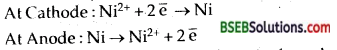Thus the amount of nickel deposited on the cathode from the solution-nickel dissolved from anode in the solution, Hence molarity of the solution will remain unchanged at the end of electrolysis.

Question 6.
In each of the following pairs, which will have greater conduction of electricity and why?
(i) Silver wire at 20°C, same silver wire at 50°C.
(ii) NH4OH solution at 20°C, same NH4OH solution at 50°C.
(iii) 0.1 M acetic acid solution 1 M acetic acid solution.
(i) Silver wire at 20°C, because conduction decreases with increase in temperature due to vibration of kernels in metals.
(ii) NH4OH at 50°C, because in the case of weak electrolytes dissociation increases with increase in temperature.
(iii) 0.1 M acetic acid because with dilution, dissociation/ ionisation increases.

Question 7.
An electrochemical cell is made of aluminium and tin electrodes with their standard reduction potentials -1.66 V and 0.14 V respectively. Select the anode and the cathode, represent the cell reaction and the cell. Find the e.m.f. of the cell. (P.S.B. 2003)
For Ecell to be positive, the given standard reduction potentials show that oxidation will take place on Al-electrode. Hence Al will be anode and Sn will be cathode. the cell will be= 0.14-(-1.66) = 1.80 V

Question 8.
Which type of cells are rechargeable?
Those cells are rechargeable in which the products formed during discharge are deposited on the electrodes and these can be decomposed to give the original substances when electrical energy from an external gource is supplied.

Question 9.
What is the role of zinc chloride (Zn Cl2) in a dry cell?
ZnCl2 combines with NH4 released from NH4Cl present in the cell to form the complex salt [Zn (NH3,)2 Cl2,]. Otherwise the pressure developed due to released NH., would crack the seal of the cell.

Question 10.
Why electrolysis of NaBr and Nal gives Br2 and I2 respectively while that of NaF gives O2 instead of F2?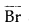and I ions have higher oxidation potentials than water. Hence they are more easilv oxidized. But F ions have lower oxidation potential than H2O. So it is 11,0 which is easily oxidized to give O2.Question 11.
State Kohlrausch’s law. How will you calculate ∧°m of Ba(OH)2 with the help of the law. (H.S.B. 2005)
Please see the text for Kohlrausch’s law.
∧°m [Ba(OH)2] = λ°Ba2+ + 2 × λ°OH
where λ°Ba2+ and λ°OH represent molar ionic conductances of
Ba2+ and OH ions.

Question 12.
The standard reduction potentials of three metallic cations X, Y and Z are 0.52, – 3.03, -1.18 V respectively. What will be the order of reducing power of the corresponding metals? (A.I.S.B. 2005 C)
The standard oxidation potentials of the metals X, Y, Z will be – 0.52, + 3.03 and + 1.18 V respectively. Higher the oxidation potential, more easily the metal is oxidized and hence greater is the reducing power. Hence the reducing powers will be in the order Y > Z > X.

Question 1.
Define KOHLRAUSCH’S LAW. How does it help in
(i) calculating ofΔ for a weak electrolyte.
(ii) degree of dissociation of a weak electrolyte.
“The molar conductivity of an electrolyte at infinite dilution is the sum of the ionic conductivities of the cations and anions each multipled with the number of ions present in one formula unit of the electrolyte.” ‘
Mathematically ∧m for AxBy = xλAy++ yλBy
where ∧m is the molar conductivity of the electrolyte at infinite dilution, xλAy+ and yλBy are the molar conductivities of the cation Ay + and the anion Bx respectively at infinite dilution.
For exampleIn term of equivalent conductivities, Kohlrausch’s law is defined as follows. “The equivalent conductivity of an electrolyte at infinite dilution is the sum of two values – one depending upon the cation and the other upon anions.”
i.e., ∧eq = λ ca
where λ c and λa are called the ionic conductivities at infinite dilution for the cation and anion respectively.

Applications of Kohlrausch’s Law-Some of the important applications of Kohlrausch’s law are :
1. Calculation of molar conductivities of weak electrolytes at infinite dilution-Kohlrausch’s law is helpful in determining the limiting molar conductivities of weak electrolytes. It has been discussed earlier ∧m the value of for weak electrolytes cannot be obtained directly by the extrapolation of plot of ∧m versus $$\sqrt{c}$$ But this can be easily calculated with the help of Kohlrausch’s law.

For example, the value of λ c for acetic acid (CH3COOH) can be calculated from the knowledge of the molar conductivities at infinite dilution of Strong electrolytes like CH3COONa, HCl and NaCl as follows
λ c (CH3COOH) = λ (CH3COO) +λ (H+)
Now, add and subtract λ Na+ and λ Cl to the expression on right hand side and rearrange.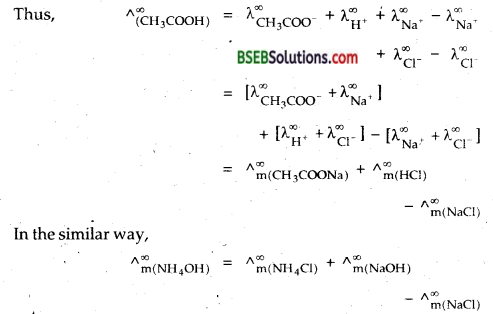2.Calculation of degree of dissociation of weak electrolyte-Molar conductivity of an electrolyte depends upon its degree of dissociation. Higher the degree of dissociation, higher is the molar conductivity. As dilution increases, the degree of dissociation of weak electrolyte also increases and consequently, molar conductivity of electrolyte increases. At infinite dilution molar conductivity becomes maximum because degree of dissociation approaches unity.
Thus,∧ mc if = molar conductivity of solution at any concentration.
m= molar conductivity at infinite dilution
α = degree of dissociation
α = $$\frac{\wedge_{\mathrm{m}}^{\mathrm{c}}}{\wedge_{\mathrm{m}}^{\infty}}$$Question 2.
(a) Distinguish between Electrolytic cell and Electrochemical cell.
(b) E.M.F. and Potential difference
(c) Metallic conduction and Electrolytic conduction*
(a) Distinction between Electrolytic and Electro chemical cell or (Galvanic cell).

 Galvanic cell Electrolyte cell 1. In galvanic cell, electrical energy is produced. 1. In electrolytic cell, electrical energy is consumed. 2. In galvanic cell, reaction taking place is spontaneous. 2. In electrolytic cell, reaction taking place is non- spontaneous. 3. The two half cells are set up in different containers and are connected through salt bridge or porous partition. 3. Both the electrodes are placed in the solution or molten electrolyte in the same container. 4. In galvanic cell, anode is negative and cathode is positive. 4. In electrolytic cell, the anode is positive and cathode is negative. 5. The electrons move from anode to cathode in external circuit. 5. The electrons are supplied by the external source. They enter through cathode and come out through anode.

(b) Distinction between Potential difference and E.M.F. of the

 Potential difference E.M.F. 1. It is the difference of potential between the two electrodes when the cell is producing current i.e., the circuit is closed. 1. Ii is the difference of potential between the two electrodes when no net current flows through the cell i.e. when the circuit is open. 2. It is always less than the e.m.f. of the cell. 2. it is the maximum potential difference between the two electrodes of the cell. 3. It does not have a definite value and varies as the cell produces electricity. 3. It has a definite value for the cell. 4. The work calculated from the potential difference is always less than the maximum work obtainable from the cell. 4. The maximum that can be obtained from a cell is calulated from its e.m.f.

(c) Distinction between Metallic conduction and Electrolytic conduction.

 Metallic Conduction Electrolytic Conduction 1. Conduction through metals is carried by the movement of electrons. 1. Conduction through electrolytes is carried by movement of ions. 2. No change is observed in the chemical properties of the conductors. 2. It involves chemical change resulting in the decomposition of electrolytes. 3. It does not involve any transfer of matter. 3. It involves the transfer of matter as ions. 4. The resistance of metals is due to obstacles of vibrating kernels of metal atoms. 4. The resistance of solution of electrolyte is due to factors like inter-ionic attraction, viscosity of solvent etc. 5. It shows increase in resistance with the increase in temperature. 5. It shows decrease in resistance with the increase in temperature. 6. The conductivity of metals is generally high. 6. The conductivity of electrolytic solutions is generally low.

Question 3.
Define an Electrochemical cell. What is its principle? Describe the working of Daniel’s cell. Describe its representation. What is the role of salt bridge? Give some other examples of cells.
A VOLTAIC/GALVANIC/ELECTROCHEMICAL cell is a divice used to convert checmical energy into electrical energy .
The underlying principle is that (-ΔrGθ) the decrease in the Gibbs free energy is a measure of electrical energy produced.
rGθ = Welectrical
= nFE°
E° = E.M.F. of the cell
n = number of electrons transformed during the reaction
= 96,500 coulombs.

One such cell is DANIEL’S CELL described below.
It is simple form, a zinc strip is dipped in the ZnSO4 solution and a copper strip is dipped in the CuSO4 solution taken in separate beakers. The two metallic strips which act as electrodes are connected by the conducting wires through a voltmeter. The two solutions are joined by an inverted U-tube known as salt bridge. The U-tube is filled with the solution of some electrolyte such as KCL, KNO3 or NF4Cl to which gelatin or agar-agar has been added to convert it into semi-solid paste. A schematic diagram of this cell has been shown in Fig. below.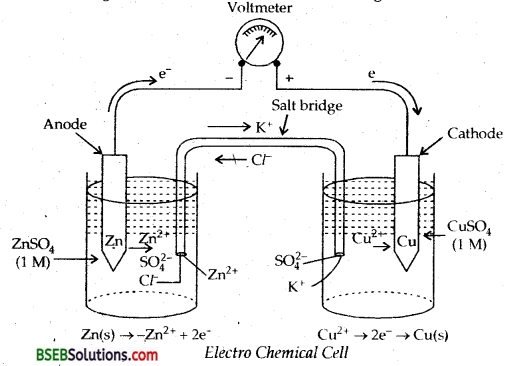The deflection in voltmeter indicates that there is a potential difference between the two electrodes. It has been found that the
conventional current flows through the outer circui t from copper to zinc strip. It implies that the electrons flow occurs from zinc to copper strip.

Let us now understand the working of the cell.
(i) Zinc undergoes oxidation to form zinc ions
Zn (s) → Zn2+ (aq) + 2e (Oxidation)
(ii) The electrons liberated during oxidation are pushed through the connecting wires to copper strip.
(iii) Copper ions mcve towards copper strip, pick up the electrons, and get reduced to copper atoms which are deposited at the copper strip.
Cu2+ (aq) + 2e → Cu (s) (Reduction)

The electrode at which oxidation occurs is anode and that at which reduction occurs is cathode. In the above cell, zinc strip is anode and copper strip is cathode. Due to the oxidation process occurring at the anode, it becomes a source of electrons and acqu ires a negative charge in the cell. Similarly, due to reduction process occurring at the cathode it acquire positive charge and becomes a receiver of the electrons. Thus, in the electrochemical cell,
Anode constitutes – ve terminal and
Cathode constitutes + ve terminal.
Function of Salt Bridge-In the electrochemical cell a salt bridge serves two very important functions:

1. Hallows the flow of current by completing the circuit.
2. It maintains electrical neutrality.

The anions of the electrolyte in the salt bridge migrate to the anode compartment and cations to the cathode compartment. Thus, the salt bridge prevents the accumulation of charges ahd maintains the flow of current. In the electr ochemical cell, the salt bridge can be replaced by the porous partition which allows the migration of ions.
Representation of Galvanic Cell—Galvanic cell is a combination , of two half cells, namely; oxidation half cell and reduction half cell. If M represents the symbol of the element and Mn+ represents its cation in solution, then
Oxidation half cell is represented as M/Mn+ (C)
Reduction half cell is represented as Mn+ (C) /M.

In both the notations C refers to the concentration of the ions in solution. Conventionally, a cell is represented by writing the cathode on the right hand side and anode on the left hand side. The two vertical lines are put between the two half cells which indicate salt bridge. Sometimes the formula of the electrolyte used in the slat bridge and is also written below the vertical lines. Some common cells along with the net cell reactions are given below in tabular form.